# Using Jax to Compute Block-Low-Rank Format Preconditioners on Graphics Cards

July 16, 2022

NOTE: Still waiting on some results will update soon! The Block-Low-Rank (BLR) representation of a matrix $$A$$ is a datasparse representation of $$A$$ which uses low-rank factors to represent off-diagonal blocks and full-rank but small dense matrices to represent its diagonal blocks. This format and preconditioners using it made a small revolution in sparse linear algebra because it provided the first general-purpose way to approximate the inverse of a sparse matrix while accounting for the fact that this inverse is almost always dense. Unfortunately the BLR format is unwieldy to code efficiently and harder on top of that to approximate a given matrix $$A$$ in this format (in spite of randomized algorithms that already exist which I will comment on later). In this blog post I convert core BLR operations (like matrix-vector product) to tensor contraction which allows a concise implementation in the jax python library. Jax converts the tensor contractions to XLA for efficent evaluation on graphics cards. A side-benefit of the Jax translation is access to algorithmic differentiation and thus we also achieve a relatively simple way to compute BLR via a stochastic-gradient-descent-like algorithm. I show here the translation to tensor contraction, jax implementation, and preconditioner results for GMRES(1) of the subsquent learned BLR representations for the inverse $$A^{-1}$$. I start with the preconditioner formulation

# Motivation for using BLR as a Preconditioner

Suppose we have an $$m \times m$$ sparse matrix $$A$$ and wish to solve the system of linear equations

\begin{align} Ax = b \end{align}

Since $$A$$ is sparse the common solution is an iterative solver, but iterative solvers generally require a preconditioner for robust and efficient convergence. For the purpose of this blog post we will consider a preconditioner $$P$$ as an approximation to the inverse of $$A$$:

\begin{align} P \approx A^{-1} \end{align}

A well-known fact about sparse matrices possessing the strong hall property is that absent numerical cancellation their inverse $$A^{-1}$$ is fully dense. Since sparse matrices tend to have extremely large dimensions $$m$$ this means a preconditioner $$P$$ has the task of approximating $$m^2$$ numbers, which is an impossible task for large $$m$$.

The BLR format compresses an otherwise intractably huge dense matrix by assuming its off-diagonal blocks have low rank. I illustrate with a $$4 \times 4$$ block matrix below

$$P \approx \begin{pmatrix} D _ 1 + U_{1,1}V_{1,1}^T & U_{1,2}V_{1,2}^T & U_{1,3}V_{1,3}^T & U_{1,3}V_{1,3}^T \\ U_{2,1}V_{2,1}^T & D _ 2 + U_{2,2}V_{2,2}^T & U_{2,2}V_{2,3}^T & U_{2,2}V_{2,3}^T \\ U_{3,1}V_{3,1}^T & U_{3,2}V_{3,2}^T & D _ 3 + U_{3,3}V_{3,3}^T & U_{3,3}V_{3,3}^T \\ U_{4,1}V_{4,1}^T & U_{4,2}V_{4,2}^T & U_{4,3}V_{4,3}^T & D _ 4 + U_{4,4}V_{4,4}^T \end{pmatrix}$$

The matrices $$U_{i,j},V_{i,j}$$ are generally tall skinny matrices representing a low-rank factorization of their corresponding off-diagonal block. By storing $$U,V$$ instead of their representative dense matrices we achieve considerable space and computational savings.

I will show later how do compute $$U_{i,j},V_{i,j},D _ i$$ later, but first will show how to compute matrix-vector products with this format efficiently. The matrix-vector operation is necessary for preconditioners and this unfortunately proves challenging to implement for the BLR format, particularly on GPUs, because it results in a significant number of small dense matrix algebra operations which must be carefully batched and fused for efficiency on modern CPUs and GPUs as well. Fortunately the Jax library can automate a lot of this for us provided we can write the algorithm under its strict limitations. I will show how to do this next.

# Implementing BLR Matrix-Vector Product as Tensor Contraction

Suppose we have the following preselected parameters:

 $$m$$ Dimension of $$A$$ $$b$$ Block size $$m _ b = m/b$$ Number of blocks $$k$$ off-diagonal rank

Then since for each $$i,j$$ $$U_{i,j},V_{i,j}\ are matrices then \(U,V$$ are 4-dimensional tensors. We may also represent an $$m \times 1$$ vector $$x$$ as a $$m _ b \times b$$ 2-dimensional tensor.

Using einstein notation we can represent the matrix-vector product $$Px$$ using jax.lax.dot_general to compute tensor contractions as follows:

import jax
from jax import grad, jit, vmap
import jax.numpy as jnp
from functools import partial

@partial(jit, static_argnums=[1,2])
def eval_blr(blocks,m,blocksize,x):
m,ncols=x.shape
nblocks=m//blocksize
Us,Vs,Ds=blocks
xr = x.reshape((nblocks,blocksize,ncols))
out=[]
for i in range(0,nblocks):
Vx = jax.lax.dot_general(Vs[i],xr,dimension_numbers=(
((2,), (1,)),
((0,), (0,))
))
UVx = jax.lax.dot_general(Us[i],Vx,dimension_numbers=(
((0,2,), (0,1)),
((), ())
))
out.append(UVx)
y=jnp.asarray(out).reshape((nblocks,blocksize,ncols))
z=y+jax.lax.dot_general(Ds,xr,dimension_numbers=(
((2,), (1,)),
((0,), (0,))
))
return z.reshape((m,ncols))


Jax is able to offload these computations to a GPU which is very useful for general speedups, but on top of this it can compute the gradient for learning the parameters $$U,V,D$$ which I show how to do next

# Learning the BLR parameters

NOTE: Check the appendix section where I will give a list of approaches which did not work. As I wrote this blog post I ran results in parallel and I would find out later that the resulting preconditioners often were no better than a standard block-Jacobi preconditioner. Weeks later I found a viable approach which delivers a preconditioner consistently better than block Jacobi. I share this successful strategy here and give a rough summary of unsuccessful strategies in the appendix.

We can learn The parameters $$U,V,D$$ through randomized algorithms (which I have linked references to below), however these algorithms have a weakness: if the dense matrix $$A ^ {-1}$$ is not well represented by the BLR format, the algorithm will fail. The reason this happens is these algorithms proceed in stages where the approximations of the previous stage are used to further approximate blocks of the next stage. If any subsequent stage fails to achieve good approximation quality the whole algorithm will fail to produce a viable preconditioner.

An alternative approach which I take here is not to proceed in stages but to learn $$U,V,D$$ simultaneously through a stochastic-gradient-descent-like algorithm. The choice of objective function to minimize by the gradient-descent algorithm is motivated by the principle of convergence for Krylov methods which suggests that eigenvalue clustering in normal operators results in faster convergence (and therefore a preconditioner which can achieve this clustering should deliver fast convergence in a Krylov method).

Computing eigenvalues of the whole operator is too slow to use in an optimization method, and furthermore would require far too much memory for bigger matrices as it ultimtately requires storing all eigenvectors associated with those eigenvalues. Instead I use the fact that the Arnoldi factorization builds up an approximation to the spectrum of the matrix $$A \in \mathbb{R}^{m\times m}$$ through

\begin{align} AV _ k = VH \end{align}

where $$V = [V_k, f] \in \mathbb{R} ^ {m \times k }$$ and $$H \in \mathbb{R} ^ {k+1 \times k}$$ is upper Hessenberg. Specifically the $$k$$ eigenvalues of the leading $$k \times k$$ square matrix of $$H$$ approximates extremal eigenvalues of $$A$$, and this approximation becomes exact if the Arnoldi method breaks down which means we have found an invariant subspace for $$A$$. This is more likely to happen when the eigenvalues of $$A$$ exhibit high clustering.

Thus to achieve high clustering I solve the following optimization problem:

\begin{align} \min _ {U,V,D} \sum _ {j = 0} ^ {k} H_{j+1,j} \end{align}

Or in words: find BLR parameters $$U,V,D$$ such that when we apply the Arnoldi factorization to the preconditioned operator $$AM$$ the residual norms at each step are as small as possible (if they are zero it signifies breakdown of the Arnoldi method, but this is very unlikely to be achieved exactly in the optimization process).

I show now how I wrote this optimization process in Python+Jax:

The Arnoldi procedure using Jax+Numpy:

#From "Templates for the solution of linear algebraic eigenvalue problems" pg. 167 (ch7 algorithm 7.6)
@partial(jit, static_argnums=[0,2])
def arnoldi_dgks(A,v,k):
norm=jnp.linalg.norm
dot=jnp.dot
eta=1.0/jnp.sqrt(2.0)

m=len(v)
V=jnp.zeros((m,k+1))
H=jnp.zeros((k+1,k))
#V[:,0]=v/norm(v)
V = V.at[:,0].set(v/norm(v))
for j in range(0,k):
w=A(V[:,j])
h=V[:,0:j+1].T @ w
f=w-V[:,0:j+1] @ h
s = V[:,0:j+1].T @ f
f = f - V[:,0:j+1] @ s
h = h + s
beta=norm(f)
#H[j+1,j]=beta
H = H.at[j+1,j].set(beta)
#V[:,j+1]=f/beta
V = V.at[:,j+1].set(f.flatten()/beta)
return V,H



The objective function:

@partial(jit, static_argnums=[1,2])
def loss(params,m,blocksize,A,b):
#Just hardcode size of arnoldi factorization for now
k=10
m,ncols=b.shape
assert(ncols==1)
#Implement the preconditioned operator
@jit
def Ac(x):
return A @ eval_blr(params,m,blocksize,x.reshape((m,ncols)))
#Construct the factorization.
#I do not need V here so I discard it.
_,H=arnoldi_dgks(Ac,b.reshape((m,)),k)
#Sum up the lower subdiagonal entries of H
return sum(H[j+1,j] for j in range(H.shape-1))


The initial condition for the optimization problem. I simply set it to be equivalent to the identity matrix

def make_blr_id(A,blocksize,d=1):
key=random.PRNGKey(0)
A=sp.lil_matrix(A)
m,_=A.shape
assert( m%blocksize==0 )
blockVs=[]
blockUs=[]
Ds=[]
for i in range(0,m,blocksize):
Us=[]
Vs=[]
ki=min(i+blocksize,m)-i
ids=list(range(i,i+ki))
Ds.append(jnp.eye(len(ids)))
for j in range(0,m,blocksize):
kj=min(j+blocksize,m)-j
Vs.append(jnp.zeros((d,kj)))
Us.append(jnp.zeros((ki,d)))
blockVs.append(jnp.asarray(Vs))
blockUs.append(jnp.asarray(Us))

return jnp.asarray(blockUs,dtype=np.float64),jnp.asarray(blockVs,dtype=np.float64),jnp.asarray(Ds,dtype=np.float64)


And finally the SGD loop where I use optax

  for it in range(nepochs):
if plot_eigs and it%10==0:
minx=min(np.real(eigA))
maxx=max(np.real(eigA))
miny=min(np.imag(eigA))
maxy=max(np.imag(eigA))
plt.close()
blrA = eval_blr(blr,m,blocksize,Afull)
eigbA = la.eigvals(blrA)
plt.scatter(np.real(eigbA),np.imag(eigbA))
ax = plt.gca()
ax.set_xlim([minx,maxx])
ax.set_ylim([miny,maxy])
istr=str(it).zfill(5)
plt.title(f"Preconditioned spectrum at SGD iteration {it}")
plt.xlabel("Real part")
plt.ylabel("Imaginary part")
plt.savefig(f"eigs/{istr}.png")

start=time.time()
err=loss(blr,m,blocksize,Aj,b)

stop=time.time()
print(f"it = {it},     elapsed = {stop-start : .4f},    loss = {err : 4f},    ")
if losses and err<min(losses):
f=open("blr_best.dat","wb")
pickle.dump(blr,f)

losses.append(err)


Note I have all this code on github but it is subject to change and very messy!

# Preconditioner Results of Learned BLR on GMRES

To see how well this functions as a preconditioner I compare it to standard block-jacobi using the same block size as the BLR preconditioner:

#For comparison purposes: block jacobi preconditioner
def make_block_precon(A,blocksize):
A=sp.lil_matrix(A)
m,_=A.shape
blocks=[]
for i in range(0,m,blocksize):
beg=i
end=min(i+blocksize,m)
ids=list(range(beg,end))
blocks.append(A[np.ix_(ids,ids)])
return sp.block_diag(blocks)



and I tested it against the following family of sparse banded matrices

#Make a random sparse-banded matrix
#with bands in bands1
#its diagonal shifted by diag
def make_banded_matrix(m,diag,bands,rng):
subdiags=[rng.uniform(-1,1,m) for _ in bands] + [rng.uniform(0.1,1,m) + diag] + [rng.uniform(-1,1,m) for _ in bands]
offs = [-x for x in bands] +  + [x for x in bands]
return sp.diags(subdiags,offs,shape=(m,m))

#Example matrix
m=1024
seed=23498732
rng=np.random.default_rng(seed)
diag=3
A=make_banded_matrix(m,diag,[1,2,3,10,40,100],rng)


The diag parameter shifts the diagonal of A and the closer diag is to 0 the harder it is to solve systems $$Ax=b$$ with iterative methods because $$A$$ becomes more indefinite, necessitating better preconditioners.

## Early Results

I chose parameters m=512,blocksize=128 so that the experiments would be able to run on my laptop. I will later run larger experiments on GCP A100 instances.

First I compare preconditioner quality by measuring the residual norm at each GMRES iteration for both the BLR preconditioner and block-jacobi based on the same blocksize.

diag GMRES Iteration History
4.03.02.01.51.0I also show how the SGD training loss looks like per iteration. It appears there is likely room for more improvement if I let the iterations proceed longer

diag Training Loss
4.03.02.01.51.0Finally I show animations for what the preconditioned opeartor $$MA$$ spectrum looks like as the SGD iterations proceed. We can see a squeezing of the spectrum to a point - this is expected because we are driving the arnoldi iterations to a breakdown scenario. Arnoldi also picks out extremal eigenvalues very well so we should expect the extremal eigenvalues to be most impacted and that is what we see here.

diag Spectrum GIF
4.0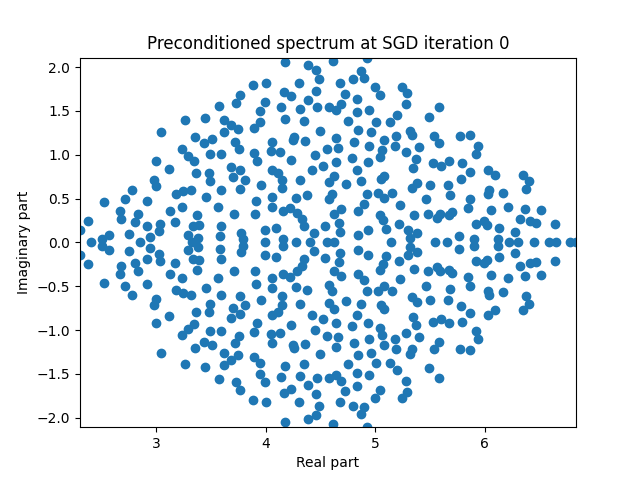3.0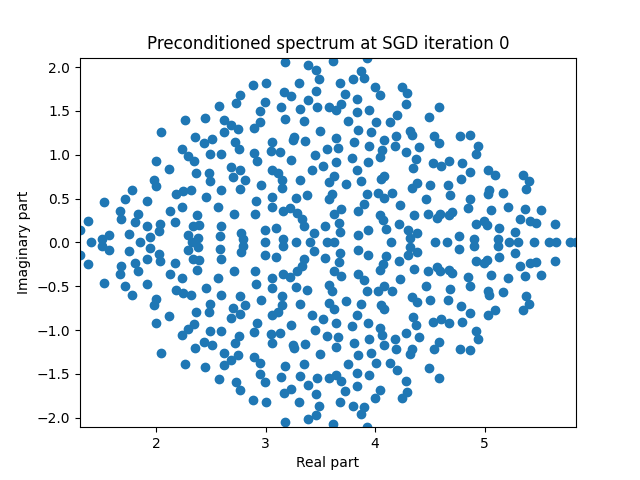2.0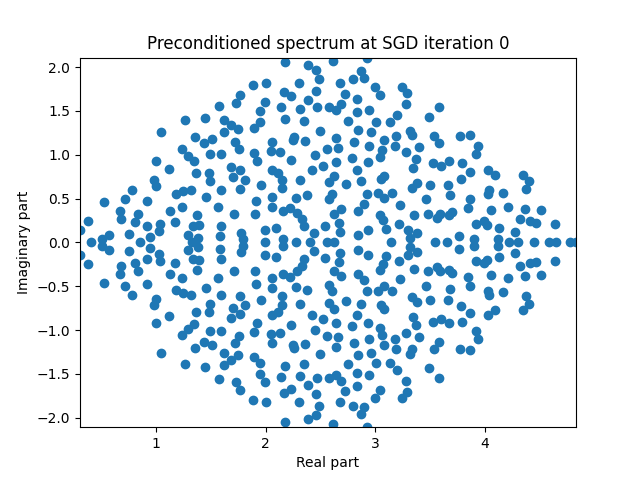1.5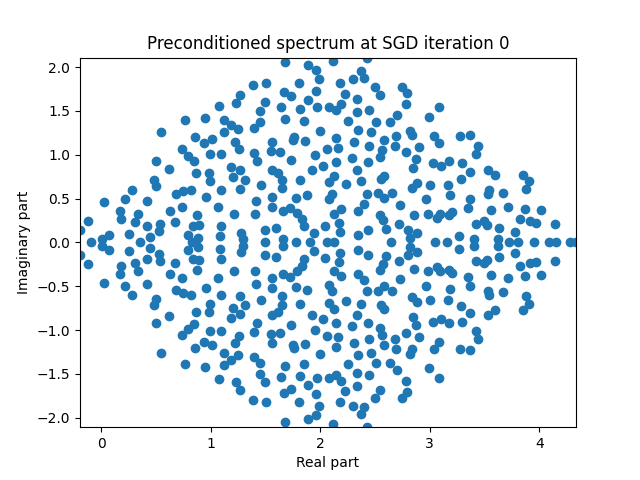1.0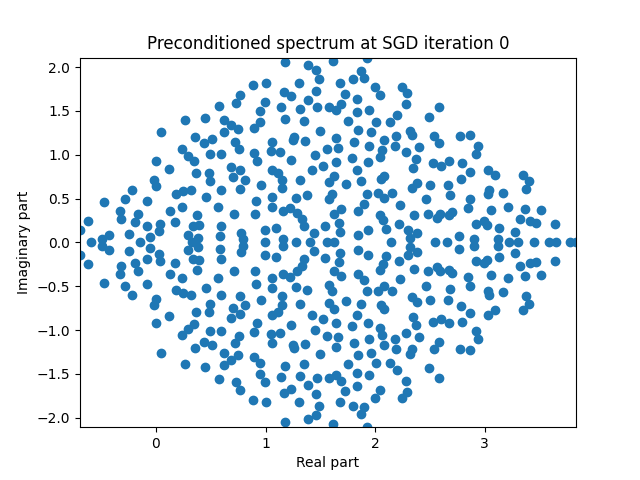The learned BLR preconditioner worked best when we had diag>=2` which corresponded to definite operators. I believe if we had a simple preconditioner which dealt only with this part of the spectrum we could combine the result with the learned BLR preconditioner. One way we could possibly approach this would be to split $$A = \frac{1}{2}(A+A^T) + \frac{1}{2}(A-A^T)$$ and compute a cholesky-like factorization for the symmetric part leaving the BLR factorization to the resulting shifted skew-symmetric system.

# Conclusions

While I do not think I have made a very useful preconditioner algorithm through the SGD training procedure I do believe that the formulation of the BLR preconditioner as tensor contractions could prove very useful for experimenting with BLR preconditioners on the GPU. The SGD algorithm could perhaps be further improved for more rapid convergence as well though, and also could be used to “refine” an existing BLR preconditioner (acheived through e.g. a randomized algorithm).

# Appendices 1: References

Datasparse formats (not just BLR) has received significant interest after Martinsson published a randomized algorithm to approximate an input matrix with a datasparse representation, an algorithm that can readily be adapted for the BLR case. The Martinsson algorithm was very interesting because it was “black box”: you did not need to know the actual entries of $$A$$ to use it, only the ability to compute matrix-vector products $$Ax$$. This enabled a new class of preconditioners which have proven extremely powerful for a wide variety of problems. I give below a small list of references which form a good starting place for learning more about these preconditioners

# Appendices 2: Failures

When I started this blog post I had several numerical experiments running for fairly large problems using GCP A100 instances. I found out mid-writing that these did not work very well. My choice of objective function at the beginning was not ideal for producing a good preconditioner for GMRES. Here is what I tried that did not seem to work:

1.  Minimizing preconditioner error: $$MA - I$$
2. Minimizing residual of a few steps of GMRES
3. Minimizing the distance of the eigenvalues of $$MA$$ to $$1$$

I believe that these did not work because although they superficially improved commonly used metrics to determine preconditioner quality, they tended to increase nonnormality of the resulting operator $$MA$$ in equal measure. This resulted in a preconditioner that looks good “on paper” but does not work as expected in practice.

The final objective function based on the arnoldi factorization avoids this problem because any non-normality of the operator $$MA$$ gets accounted for in the factorization, which evaluates a polynomial of the matrix $$MA$$.